## Monday, July 18, 2016

### Power BI/PowerPivot: What is Row Context?

Today, we're going to talk about another major component of DAX, Row Context.  In a previous post, we talked about Filter Context, which is supplied by charts, slicers, and measures at runtime.  Row Context is the complement to Filter Context.  Row Context is used when you are creating calculated columns within your data model.  This means that they are calculated at processing time instead of at run time.  Let's start with a simple data model.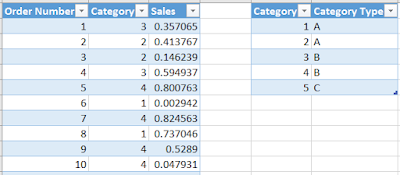Sales DataData Model
We have a sales table with a row-level slicer called [Order Number] and a look-up slicer called [Category].  We also have a simple relationship between the Sales and Categories table on the [Category] column.  Let's start by adding a very simple calculated column to the Sales table.Category 1?
This column simply returns TRUE if Sales[Category] = 1, and FALSE otherwise.  For each row in this table, the [Category 1?] calculation has a Row Context defining what row it is on.  While working within a single table without an iterator, Row Context is quite simple.  It's nothing more than the row you are on.  There are a few ways where this can get sticky.

Retrieving Values from UP the Hierarchy

Let's start by looking UP the hierarchy, i.e. from the Sales table up to the Categories table.Category Type (Invalid)
If we try to simply pull the value down, we get an error.  This is because, unlike Filter Context, Row Context does not move across relationships.  We have to use specialized functions to accomplish certain tasks.  If we want to pull down a slicer like this, we need to use the RELATED() function.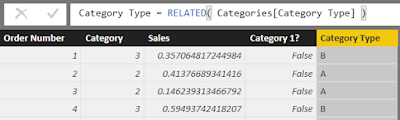Category Type (Related)
The RELATED() function is able to pass Row Context up the hierarchy, allowing you to retrieve values from lookup tables.  This is one of the more common ways that we use to pull data across tables.

Retrieving Values from DOWN the Hierarchy

Next, let's try to pull a value from DOWN the hierarchy, i.e. from the Categories table down to the Sales table.  Since we have multiple rows in the Sales table related to a single row in the Categories table, we're going to need to aggregate the values.  Let's try to pull up Total Sales.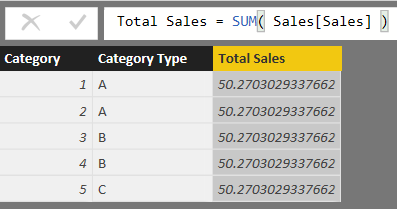Total Sales (Invalid)
Well, this is interesting.  When we first started using DAX, we always assumed that using calculations like this would work just like a pivot table.  If we built a pivot table with Category and Category Type as the columns and SUM( Sales[Sales] ) as the measure, this would work.  However, we now know that pivot tables work off Filter Context, while calculated columns work off Row Context.  It's important to remember that Filter Context can move across relationships, while Row Context cannot.  So, we need a way to turn this Row Context into Filter Context.  This concept is called "Context Transition".  Luckily, this is one of the many uses of the CALCULATE() function.Total Sales (Calculate)
Voila!  The answer magically appears.  Well, not magically, but it's still pretty cool.  Let's move on to the final method.

Iterators

Iterators are a huge category of functions in DAX that can be used to solve almost any problem.  We could easily do an entire blog series on iterators.  So, we're just going to touch on a very simple iterator to showcase a little more about Row Context.  Let's say that you wanted to create a new column in your table for Running Sum of Sales.  You can order this running sum however you want.  For our purposes, we'll order it by Sales[Order Number].  This means that [Running Sum of Sales] for Order Number 1 is just the sales of Order 1.  The [Running Sum of Sales] for Order Number 2 is the sum of Order Number 1 and Order Number 2's Sales.

Before we can start looking at other rows, we need to be able to escape the Row Context of our current row.  We can accomplish this with the ALL() function.  The ALL() function simply removes all filters applied to a calculation.  Remember that once we wrap our calculation in the CALCULATE() statement, our Row Context becomes Filter Context, meaning that it can be escaped using ALL().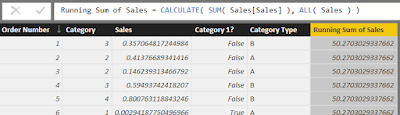Running Sum of Sales (Incomplete)
We can see that we've removed all Row (and Filter) Context from this calculation.  Next, we need to add more logic so that it only looks at rows with Order Number less than or equal to the Order Number of the current row.  But, how do we access the Row Context of the current row now that we've removed it.  This is where the EARLIER() function comes in handy.  The EARLIER() function can look back at what your Row Context used to be.  This means that we create a filter statement using this original Row Context.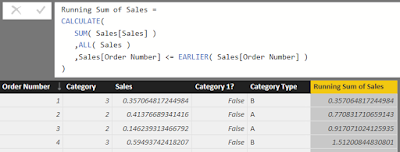Running Sum of Sales
Now, our calculation is complete.

Hopefully, learning about Row Context has opened your eyes to some of the more interesting possibilities within DAX.  Keep your eyes peeled for more posts on the amazing world of DAX.  Thanks for reading.  We hope you found this informative.